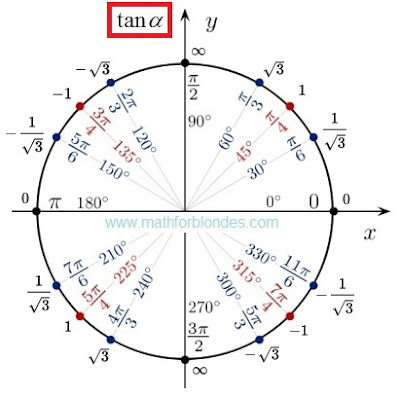## 2.10.2017

### Trigonometrical circle of a tangent

Last time I drew for you a unit circle of cotangent. Now you can look at a trigonometrical circle of tangent.Trigonometrical circle of a tangent
There is no sense here. There are angles and values of tangents. If you want to understand sense of a tangent and cotangent, look here.

1.Here is deep description about the article matter which helped me more. unit circle

2.At the top and bottom it should be DNE.

1.Does Not Exist... If we do not understand how to divide by zero, this does not mean that division by zero is impossible. I will tell you what it is. But for this you need to know a little more mathematics.